## Selina Concise Mathematics Class 10 ICSE Solutions Chapter 14 Equation of a Line Ex 14C

These Solutions are part of Selina Concise Mathematics Class 10 ICSE Solutions. Here we have given Selina Concise Mathematics Class 10 ICSE Solutions Chapter 14 Equation of a Line Ex 14C.

Other Exercises

Question 1.
Find the equation of line whose :
(i) y-intercept = 2 and slope = 3,
(ii) y-intercept = -1 and slope = $$\frac { -3 }{ 4 }$$
Solution:
(i) The point whose y-intercept = 2 will be (0, 2) and slope (m) = 3.
Equation of line will be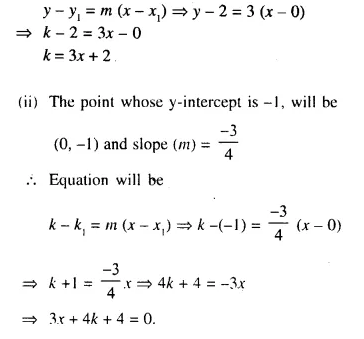Question 2.
Find the equation of a line whose :
(i) y-intercept = -1 and inclination = 45°
(ii) y-intercept = 3 and inclination = 30°
Solution:
(i) The point whose y-intercept is -1, will be (0, -1) and inclination = 45°
Slope (m) = tan 45° = 1
Equation will beQuestion 3.
Find the equation of the line whose slope is $$\frac { -4 }{ 3 }$$ and which passes through (-3, 4).
Solution:
Slope of the line (m) = $$\frac { -4 }{ 3 }$$
The point from which the line passes (-3, 4)
Equation of line will be y – y1 = m (x – x1)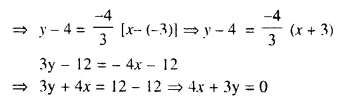Question 4.
Find the equation of a line which passes through (5, 4) and makes an angle of 60° with the positive direction of the x-axis.
Solution:
The line passes through the point (5, 4) and angle of inclination = 60°
slope (m) = tan 60° = √3
Equation of line
y – y1 = m (x – x1)
⇒ y – 4 = √3 (x – 5)
⇒ y – 4 = √3 x – 5√3
⇒ y = √3 x + 4 – 5√3

Question 5.
Find the equation of the line passing through:
(i) (0, 1) and (1, 2)
(ii) (-1, -4) and (3, 0)
(iii) (4, -2) and (5, 2)
Solution:
Two given points are (0, 1) and (1, 2)
Slope of the line passing through these two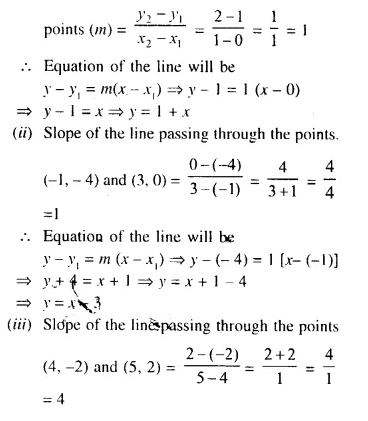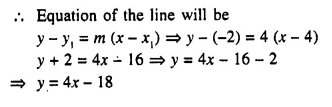Question 6.
The co-ordinates of two points P and Q are (2, 6) and (-3, 5) respectively. Find :
(ii) The equation of PQ,
(iii) The co-ordinates of the point where PQ intersects the x-axis.
Solution:
Two points P (2,-6) and Q (-3, 5) are given.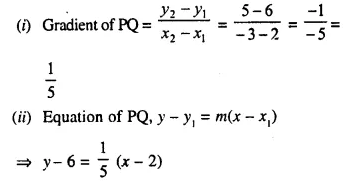⇒ 5y – 30 = x – 2
⇒ 5y = x – 2 + 30
⇒ 5y = x + 28 ….(i)
(iii) Co-ordinates of the point where PQ intersects x-axis will be = 0
substituting, the value of y in (i)
5 x 0 = x + 28 ⇒ x + 28 = 0 ⇒ x = -28
Co-ordinates of point are (-28, 0)

Question 7.
The co-ordinates of two points A and B are (-3, 4) and (2, -1). Find :
(i) the equation of AB
(ii) the co-ordinates of the point where the line AB intersects they- axis.
Solution:
Slope of the line passing through two points A (-3, 4) and B (2, -1) will be :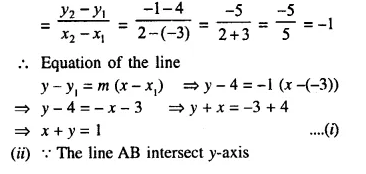Its abscissa = 0
substituting, the value of x = 0 in (i)
0 + y = 1
y = 1
Co-ordinates of point = (0, 1)

Question 8.
The figure given below shows two straight lines AB and CD intersecting each other at point P (3, 4). Find the equations of AB and CD.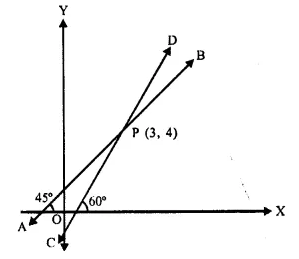Solution:
Two lines AB and CD intersect each other at P (3, 4)
AB inclined at angle of 45° and CD at angle of 60° with the x-axis.
Slope of AB = tan 45° = 1
and slope of CD = tan 60° = √3
Now, equation of line AB will be
y – y1 = m (x – x1)
⇒ y – 4 = 1 (x – 3)
⇒ y – 4 = x – 3
⇒ y = x – 3 + 4
⇒ y = x + 1
(ii) Equation of CD will be :
y – y1 = m (x – x1)
⇒ y – 4 = √3 (x – 3)
⇒ y – 4 = √3 x – 3√3
⇒ y = √3 x – 3√3 + 4
⇒ y = √3 x + 4 – 3√3

Question 9.
In ΔABC, A (3, 5), B (7, 8) and C (1, -10). Find the equation of the median through A.
Solution:
D is mid point of BC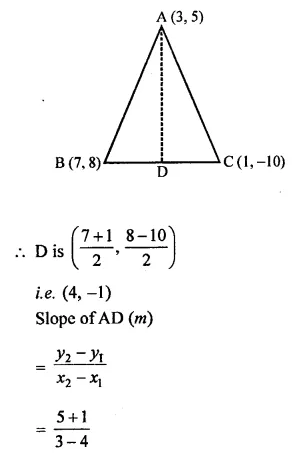y – y1 = m (x – x1)
⇒ y + 1 = -6 (x – 4)
⇒ y + 1 = -6x + 24
⇒ y + 6x = -1 + 24
⇒ 6x + y = 23

Question 10.
The following figure shows a parallelogram ABCD whose side AB is parallel to the x-axis, ∠A = 60° and vertex, C = (7, 5). Find the equations of BC and CD.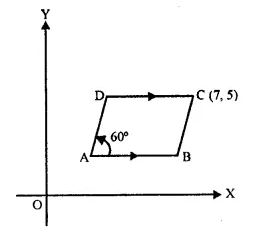Solution: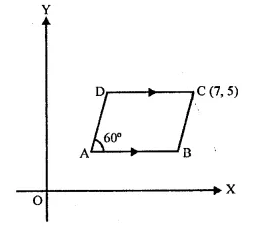ABCD is a ||gm in which AB = CD || x-axis
∠A = 60° and C (7, 5)
(i) CD || AB || x-axis ,
Equation of CD will be
y – y1 = m (x – x1)
⇒ y – 5 = 0 (x – 7)
⇒ y – 5 = 0
⇒ y = 5
Slope of BC = tan 60° = √3
Equation of BC will be
y – y1 = m (x – x1)
⇒ y – 6 = √3 (x – 7)
⇒ y – 6 = √3 x – 7√3
⇒ y = √3 x + 6 – 7√3

Question 11.
Find the equation of the straight line passing through origin and the point of intersection of the lines x + 2y = 7 and x – y = 4.
Solution:
Point of intersection of two lines
x + 2y = 7 ….(i)
x – y = 4 ….(ii)
Subtracting, we get
3y = 3
y =1
Substituting, the value of y in (ii)
x – 1 = 4
⇒ x = 4 + 1 = 5
Point of intersection is (5, 1)
Slope of the line passing through origin (0, 0) and (5, 1)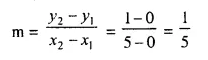Equation of line will be
y – y1 = m (x – x1)
⇒ y – 5 = $$\frac { 1 }{ 2 }$$ (x – 1)
⇒ 5y – 25 = x – 1
⇒ 5y = x – 1 + 25 = x + 24
⇒ 5y = x + 24

Question 12.
In triangle ABC, the co-ordinates of vertices A, B and C are (4, 7), (-2, 3) and (0, 1) respectively. Find the equation of median through vertex A. Also, find the equation of the line through vertex B and parallel to AC.
Solution: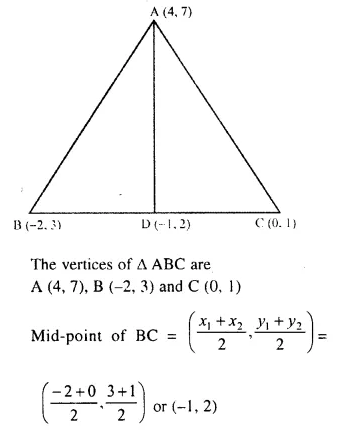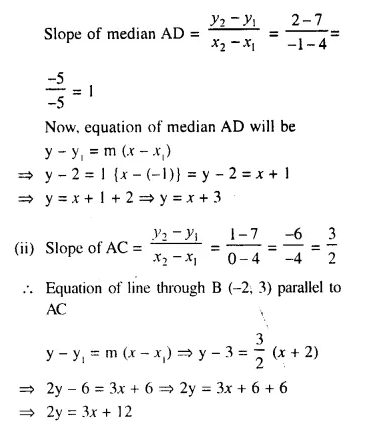Question 13.
A, B and C have co-ordinates (0, 3), (4, 4) and (8, 0) respectively. Find the equation of the line through A and perpendicular to BC.
Solution: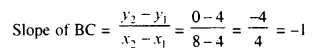Slope of line through A, perpendicular to BC = -(-1) = 1
Now, the equation of line through A (0, 3) is
y – y1 = m (x – x1)
y – 3 = 1 (x – 0)
⇒ y – 3 = x
⇒ y = x + 3

Question 14.
Find the equation of the perpendicular dropped from the point (-1, 2) onto the line joining the points (1, 4) and (2, 3).
Solution:
Slope of the line joining the points (1, 4) and (2, 3)Slope of line perpendicular to the above line = 1
Equation of line passing through (-1, 2)
y – y1 = m (x – x1)
⇒ y – 2 = 1 [x -(-1)]
⇒ y – 2 = x + 1
⇒ y = x + 1 + 2
⇒ y = x + 3

Question 15.
Find the equation of the line, whose :
(i) x-intercept = 5 and y-intercept = 3
(ii) x-intercept = -4 and y-intercept = 6
(iii) x-intercept = -8 and y-intercept = -4
(iv) x-intercept = 3 and y-intercept = -6
Solution:
(i) When x-intercept = 5, then point will be (5, 0)
and when y-intercept = 3, then point will be (0, 3)
Slope of the line passing through these points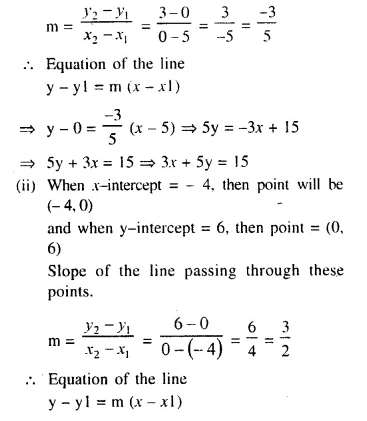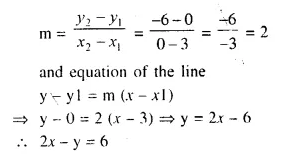Question 16.
Find the equation of the line whose slope is $$\frac { -5 }{ 6 }$$ and x-intercept is 6.
Solution: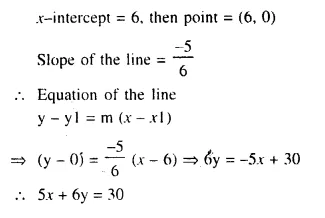Question 17.
Find the equation of the line with x-intercept 5 and a point on it (-3, 2).
Solution:
x-intercept of the line = 5
Point = (5, 0)
Slope of the line passing through the point (-3, 2)Question 18.
Find the equation of the line through (1, 3) and making an intercept of 5 on the y- axis.
Solution:
The line makes y-intercept = 5
Point = (0, 5)
Slope of the line passing through the point (1, 3) and (0, 5)Equation of the line
y – y1 = m (x – x1)
⇒ y – 3 = -2 (x – 1)
⇒ y – 3 = -2x + 2
⇒ 2x + y = 2 + 3
⇒ 2x + y = 5

Question 19.
Find the equations of the lines passing through point (-2, 0) and equally inclined to the co-ordinate axes.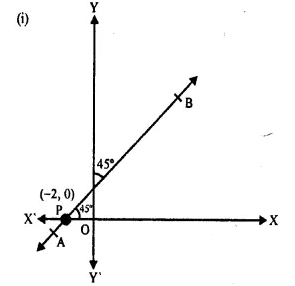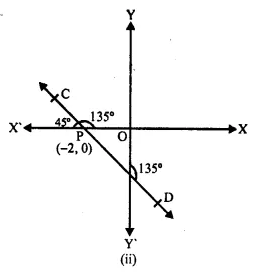Solution:
(i) Slope of line AB = tan 45° = 1
Equation passing through the point (-2, 0)
y – y1 = m (x – x1)
⇒ y – 0 = 1 (x + 2)
⇒ y = x + 2
⇒ x – y + 2 = 0
(ii) Slope of line CD = tan (-45°) = -1
Equation passing through the point (-2, 0)
y – y1 = m (x – x1)
⇒ y – 0 = -1 (x + 2)
⇒ y = -x – 2
⇒ y + x + 2 = 0
⇒ x + y + 2 = 0

Question 20.
The line through P (5, 3) intersects y axis at Q.(i) Write the slope of the line.
(ii) Write the equation of the line.
(iii) Find the co-ordinates of Q.
Solution:
(i) Here θ = 45°
So, slope of the line = tanθ = tan 45° = 1
(ii) Equation of the line through P and Q is
y – 3 = 1 (x – 5)
⇒ y – x + 2 = 0
(iii) Let the coordinates of Q be (0, y)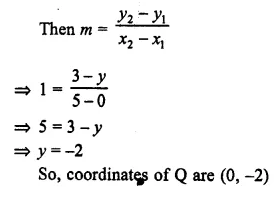Question 21.
Write down the equation of the line whose gradient is $$\frac { -2 }{ 5 }$$ and which passes through point P, where P divides the line segment joining A (4, -8) and B (12, 0) in the ratio 3 : 1.
Solution:
Slope of the line m = $$\frac { -2 }{ 5 }$$
P divides the line AB, whose co-ordinates are (4, -8) and (12, 0) in the ratio of 3 : 1
Co-ordinates of P be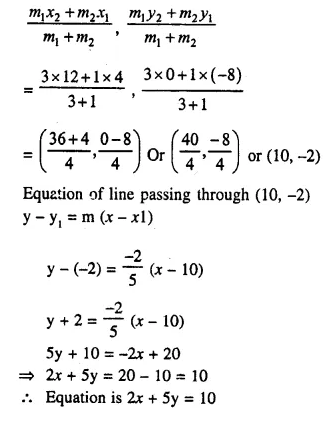Question 22.
A (1, 4), B (3, 2) and C (7, 5) are vertices of a triangle ABC. Find :
(i) the co-ordinates of the centroid of ΔABC.
(ii) the equation of a line, through the centroid and parallel to AB. 
Solution:
(i) Co-ordinates of vertices of ΔABC are A (1, 4), B (3, 2), C (7, 5)
and let G be the centroid of ΔABC.
Co-ordinates of G are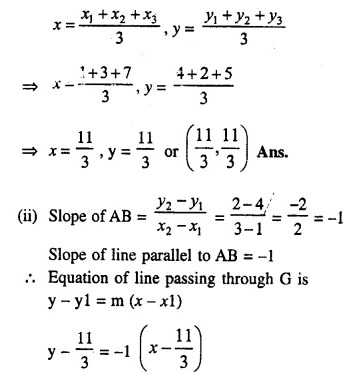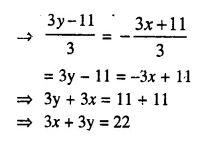Question 23.
A (7, -1), B (4, 1) and C (-3, 4) are the vertices of a triangle ABC. Find the equation of a line through the vertex B and the point F in AC; such that AP : CP = 2 : 3.
Solution:
P divides AC in the ratio of 2 : 3Hope given Selina Concise Mathematics Class 10 ICSE Solutions Chapter 14 Equation of a Line Ex 14C are helpful to complete your math homework.

If you have any doubts, please comment below. Learn Insta try to provide online math tutoring for you.